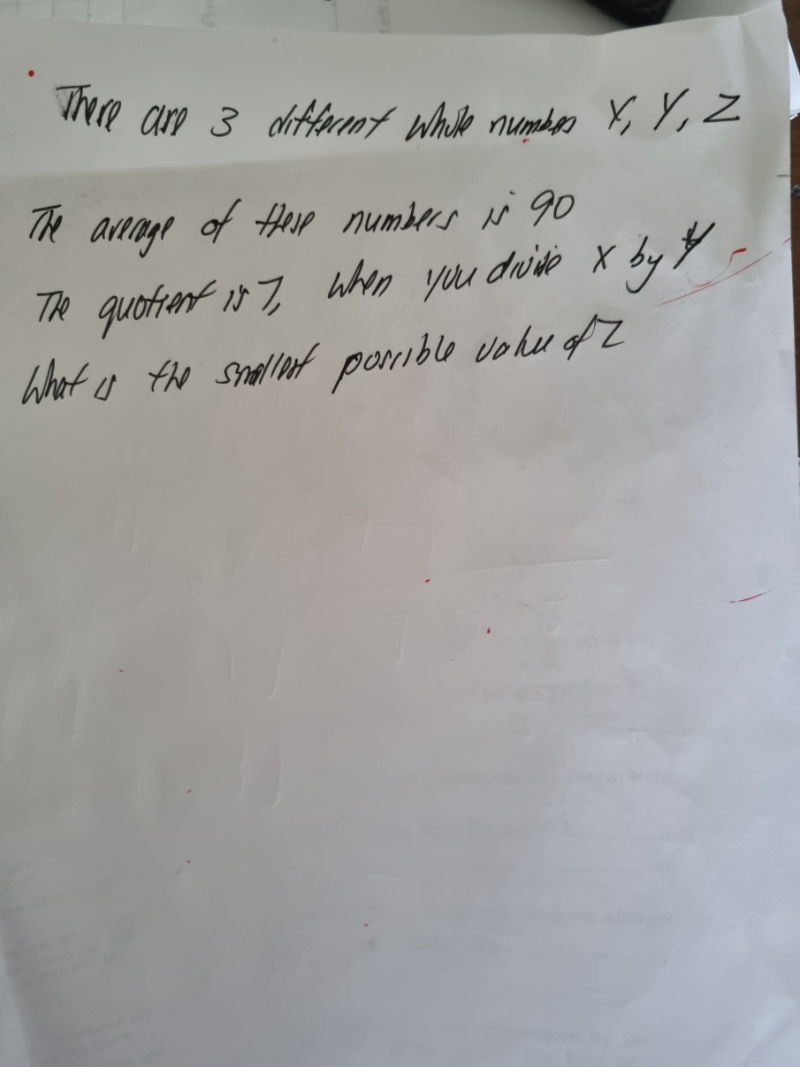# QuestionThank you .

x + y + z  = 90 x 3 = 270

x/y = 7 => x = 7y

7y + y + z = 270

z = 270 – 8y

The maximum value of 8y must be less than 270, hence 8y = 264 => y = 33   (you know 30 x 8 = 240 and 35 x 8 = 280, so y is a number between 30-35)

Therefore smallest possible value of  z = 270 – 264 = 6

Check:

7(33) + 33 + 6 = 231 + 33 + 6 = 270